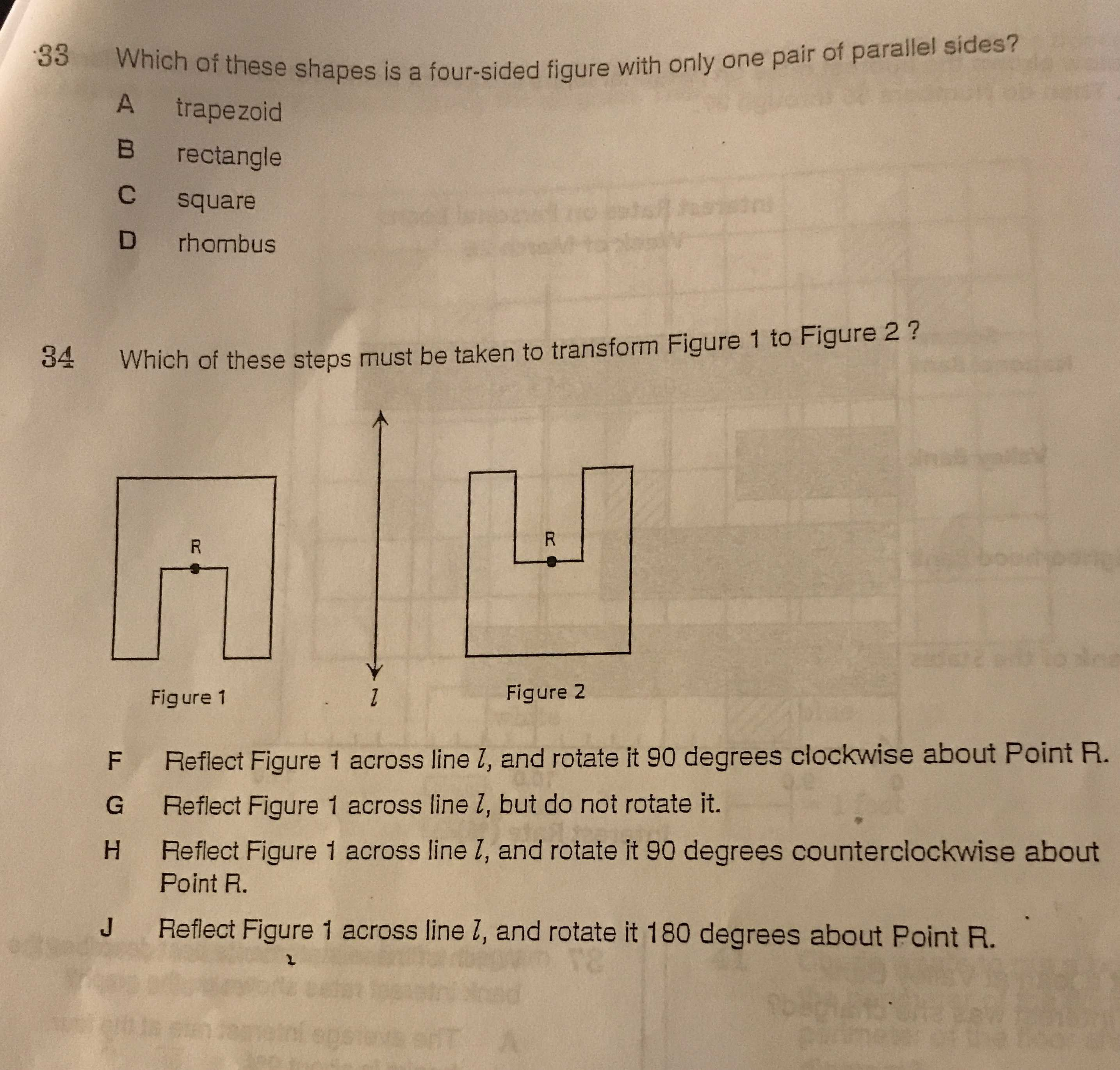### Still have math questions?

Arithmetic
Question$$33$$ Which of these shapes is a four-sided figure with only one pair of parallel sides?

A trapezoid

B rectangle

C square

D rhombus

34.Which of these steps must be taken to transform Figure $$1$$ to Figure $$2$$ ?

Reflect Figure $$1$$ across line $$l$$ , and rotate it $$90$$ degrees clockwise about Point R.

Reflect Figure $$1$$ across line $$l$$ , but do not rotate it.

Reflect Figure $$1$$ across line $$l$$ , and rotate it $$90$$ degrees counterclockwise about Point R.

Reflect Figure $$1$$ across line $$l$$ , and rotate it $$180$$ degrees about Point $$R$$ .